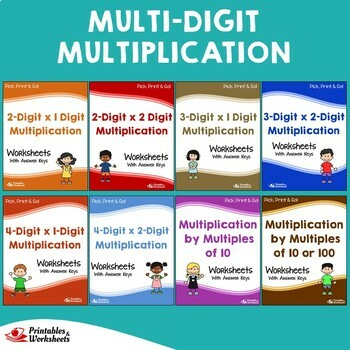# Math Fluency Multiplication And Division Worksheets Practice, AssessmentSubject
Resource Type
Product Rating
File Type

Compressed Zip File

47 MB|1000+
Share
Product Description
This is a bundle of our multiplication and division worksheets with answer keys. It includes several types of practice worksheets to help your students learn to multiply and divide numbers.

The packets are grouped by the digits used, each having multiple sets included. Just pick and print the right version for your class, and you're ready to go!

A. Multiplication Facts

> Factors of 2, 3, 4 or 5
> Factors of 6, 7, 8, 9 or 10
> Factors between 2-10
> Multiplying Numbers by 10

Sets Included:

Set 1: Multiplying Numbers – Vertical
Set 2: Multiplying Numbers – Horizontal
Set 3: Find the Missing Factor
Set 4: Find the Missing Factor or Product
Set 5: Multiplication Wheel
Set 6: Multiplication Array, Repeated Addition
Set 7: Multiplication Array, Color the Array
Set 8: Multiplication on a Number Line – Skip Count
Set 9: Use the Number Line to Multiply
Set 10: Multiplying Numbers in Words

B. Multi-Digit Multiplication

> 2-Digit x 1-Digit
> 2-Digit x 2-Digit
> 3-Digit x 1-Digit
> 3-Digit x 2-Digit
> 4-Digit x 1-Digit
> 4-Digit x 2-Digit
> Multiplying by Multiples of 10
> Multiplying by Multiples of 10 or 100

Sets Included:

Set 1: Multiplying Numbers – Vertical
Set 2: Multiplying Numbers – Vertical, Boxed Digits
Set 3: Using Grid to Multiply Numbers
Set 4: Color the Correct Answer
Set 5: Fill in the Blanks – Find the Product
Set 6: Fill in the Blanks – Find the Missing Factor
Set 7: Fill in the Blanks – Find the Missing Factor / Product
Set 8: Choose the Correct Answer from the List
Set 9: Match Two Columns
Set 10: Multiplying Numbers in Words
Set 11: Show Solution – Solve for the Product
Set 12: Show Solution – Solve for the Missing Factor
Set 13: Show Solution – Solve for the Missing Factor / Product

C. Division Facts
> Dividing numbers by 2-5
> Dividing numbers by 6-10
> Dividing by 2-10

Sets Included:

Set 1: Dividing Numbers – Vertical
Set 2: Dividing Numbers – Horizontal
Set 3: Find the Missing Divisor or Quotient
Set 4: Find the Missing Dividend, Divisor or Quotient
Set 5: Division Wheel
Set 6: Division on a Number Line – Skip Count
Set 7: Use the Number Line to Divide Numbers
Set 8: Dividing Numbers in Words

D. Multi-Digit Division / Long Division
> Dividing 2-Digit / 1 Digit Numbers
> Dividing 3-Digit / 1 Digit Numbers
> Dividing 3-Digit / 2 Digit Numbers
> Dividing 4-Digit / 1 Digit Numbers
> Dividing 4-Digit / 2 Digit Numbers

Each problem above include:
- Exact Division (No Remainder)
- Division with Remainder
- Mixed Problems - With and Without Remainders

Sets Included:

Set 1: Dividing Numbers – Vertical
Set 2: Dividing Numbers – Vertical, Boxed Digits
Set 3: Use the Grid to Divide the Numbers
Set 4: Color the Correct Answer
Set 5: Fill in the Blanks – Find the Quotient
Set 6: Fill in the Blanks – Missing Dividend
Set 7: Fill in the Blanks – Missing Number
Set 8: Choose Answers from a List
Set 9: Match the Two Columns
Set 10: Division in Words
Set 11: Show Solution – Find the Quotient
Set 12: Show Solution – Missing Dividend
Set 13: Show Solution – Missing Number

_____________________

id_3470
Total Pages
1000+